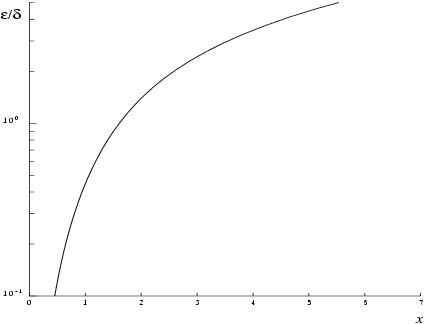NAG Library Function Document

1Purpose

nag_bessel_i0 (s18aec) returns the value of the modified Bessel function ${I}_{0}\left(x\right)$.

2Specification

 #include #include
 double nag_bessel_i0 (double x, NagError *fail)

3Description

nag_bessel_i0 (s18aec) evaluates an approximation to the modified Bessel function of the first kind ${I}_{0}\left(x\right)$.
Note:  ${I}_{0}\left(-x\right)={I}_{0}\left(x\right)$, so the approximation need only consider $x\ge 0$.
The function is based on three Chebyshev expansions:
For $0,
 $I0x=ex∑′r=0arTrt, where ​ t=2 x4 -1.$
For $4,
 $I0x=ex∑′r=0brTrt, where ​ t=x-84.$
For $x>12$,
 $I0x=exx ∑′r=0crTrt, where ​ t=2 12x -1.$
For small $x$, ${I}_{0}\left(x\right)\simeq 1$. This approximation is used when $x$ is sufficiently small for the result to be correct to machine precision.
For large $x$, the function must fail because of the danger of overflow in calculating ${e}^{x}$.
Abramowitz M and Stegun I A (1972) Handbook of Mathematical Functions (3rd Edition) Dover Publications

5Arguments

1:    $\mathbf{x}$doubleInput
On entry: the argument $x$ of the function.
2:    $\mathbf{fail}$NagError *Input/Output
The NAG error argument (see Section 3.7 in How to Use the NAG Library and its Documentation).

6Error Indicators and Warnings

NE_ALLOC_FAIL
Dynamic memory allocation failed.
See Section 2.3.1.2 in How to Use the NAG Library and its Documentation for further information.
NE_INTERNAL_ERROR
An internal error has occurred in this function. Check the function call and any array sizes. If the call is correct then please contact NAG for assistance.
See Section 2.7.6 in How to Use the NAG Library and its Documentation for further information.
NE_NO_LICENCE
Your licence key may have expired or may not have been installed correctly.
See Section 2.7.5 in How to Use the NAG Library and its Documentation for further information.
NE_REAL_ARG_GT
On entry, ${\mathbf{x}}=〈\mathit{\text{value}}〉$.
Constraint: $\left|{\mathbf{x}}\right|\le 〈\mathit{\text{value}}〉$.
$\left|{\mathbf{x}}\right|$ is too large and the function returns the approximate value of ${I}_{0}\left(x\right)$ at the nearest valid argument.

7Accuracy

Let $\delta$ and $\epsilon$ be the relative errors in the argument and result respectively.
If $\delta$ is somewhat larger than the machine precision (i.e., if $\delta$ is due to data errors etc.), then $\epsilon$ and $\delta$ are approximately related by:
 $ε≃ x I1x I0 x δ.$
Figure 1 shows the behaviour of the error amplification factor
 $xI1x I0x .$Figure 1
However if $\delta$ is of the same order as machine precision, then rounding errors could make $\epsilon$ slightly larger than the above relation predicts.
For small $x$ the amplification factor is approximately $\frac{{x}^{2}}{2}$, which implies strong attenuation of the error, but in general $\epsilon$ can never be less than the machine precision.
For large $x$, $\epsilon \simeq x\delta$ and we have strong amplification of errors. However the function must fail for quite moderate values of $x$, because ${I}_{0}\left(x\right)$ would overflow; hence in practice the loss of accuracy for large $x$ is not excessive. Note that for large $x$ the errors will be dominated by those of the standard function exp.

8Parallelism and Performance

nag_bessel_i0 (s18aec) is not threaded in any implementation.

None.

10Example

This example reads values of the argument $x$ from a file, evaluates the function at each value of $x$ and prints the results.

10.1Program Text

Program Text (s18aece.c)

10.2Program Data

Program Data (s18aece.d)

10.3Program Results

Program Results (s18aece.r)

© The Numerical Algorithms Group Ltd, Oxford, UK. 2017